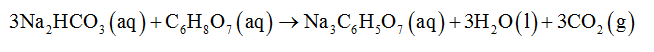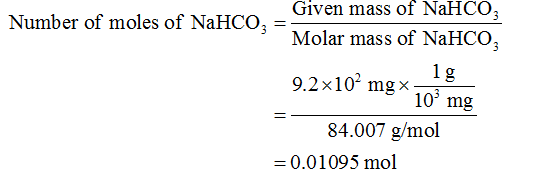# Elixirs such as Alka-Seltzer use the reaction of sodium bicarbonate with citric acid in aqueous solution to produce a fizz:3NaHCO3(aq) + C6H8O7(aq) -> 3CO2(g) +3H20(l) +Na3C6H5O7(aq)a.) What mass of C6H8O7 should be used for every 9.2 x102 mg NaHCO3?b.) What mass of CO2(g) could be produced from such a mixture?

Question
6 views

Elixirs such as Alka-Seltzer use the reaction of sodium bicarbonate with citric acid in aqueous solution to produce a fizz:

3NaHCO3(aq) + C6H8O7(aq) -> 3CO2(g) +3H20(l) +Na3C6H5O7(aq)

a.) What mass of C6H8O7 should be used for every 9.2 x102 mg NaHCO3?

b.) What mass of CO2(g) could be produced from such a mixture?

check_circle

Step 1

Mass of NaHCO3 = 9.2×102 mg

The balanced chemical equation for the reaction of sodium bicarbonate with citric acid will be represented as follows:Step 2

a. To calculate the mass of the citric first number of moles of NaHCO3 will be calculated as follows:Step 3

According to the reaction, 3 moles of sodium carbonate reacts with 1 mole of citric acid. Therefore, 0.01095 moles of sodium carbonate would react with (0.01095/3 = 0.00365)  moles of citric acid.

Thus, the mass of citric acid can be calculated as follow...

### Want to see the full answer?

See Solution

#### Want to see this answer and more?

Solutions are written by subject experts who are available 24/7. Questions are typically answered within 1 hour.*

See Solution
*Response times may vary by subject and question.
Tagged in

### Chemistry